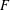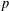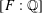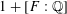Home
Hostname: page-component-544b6db54f-zts5g Total loading time: 0.21 Render date: 2021-10-23T09:19:11.182Z Has data issue: true Feature Flags: { "shouldUseShareProductTool": true, "shouldUseHypothesis": true, "isUnsiloEnabled": true, "metricsAbstractViews": false, "figures": true, "newCiteModal": false, "newCitedByModal": true, "newEcommerce": true, "newUsageEvents": true }Forum of Mathematics, Sigma

## Abstract

HTML view is not available for this content. However, as you have access to this content, a full PDF is available via the ‘Save PDF’ action button.

Let$F$ be a totally real field and let$p$ be an odd prime which is totally split in$F$. We define and study one-dimensional ‘partial’ eigenvarieties interpolating Hilbert modular forms over$F$ with weight varying only at a single place$v$ above$p$. For these eigenvarieties, we show that methods developed by Liu, Wan and Xiao apply and deduce that, over a boundary annulus in weight space of sufficiently small radius, the partial eigenvarieties decompose as a disjoint union of components which are finite over weight space. We apply this result to prove the parity version of the Bloch–Kato conjecture for finite slope Hilbert modular forms with trivial central character (with a technical assumption if$[F:\mathbb{Q}]$ is odd), by reducing to the case of parallel weight$2$. As another consequence of our results on partial eigenvarieties, we show, still under the assumption that$p$ is totally split in$F$, that the ‘full’ (dimension$1+[F:\mathbb{Q}]$) cuspidal Hilbert modular eigenvariety has the property that many (all, if$[F:\mathbb{Q}]$ is even) irreducible components contain a classical point with noncritical slopes and parallel weight$2$ (with some character at$p$ whose conductor can be explicitly bounded), or any other algebraic weight.

## MSC classification

Type
Research Article
Information
Creative CommonsThis is an Open Access article, distributed under the terms of the Creative Commons Attribution licence (http://creativecommons.org/licenses/by/4.0/), which permits unrestricted re-use, distribution, and reproduction in any medium, provided the original work is properly cited.

## References

Barrera, D., Dimitrov, M. and Jorza, A., ‘$p$ -adic$L$ -functions of Hilbert cusp forms and the trivial zero conjecture’. ArXiv e-prints, September 2017.CrossRefGoogle Scholar
Bellaïche, J. and Chenevier, G., ‘Families of Galois representations and Selmer groups’, Astérisque 324 (2009), xii+314.Google Scholar
Berger, L., ‘Équations différentielles p-adiques et (𝜙, N)-modules filtrés’, Astérisque 319 (2008), 1338. Représentations$p$ -adiques de groupes$p$ -adiques. I. Représentations galoisiennes et$(\unicode[STIX]{x1D719},\unicode[STIX]{x1D6E4})$ -modules.Google Scholar
Berger, L. and Colmez, P., ‘Familles de représentations de de Rham et monodromie p-adique’, Astérisque 319 (2008), 303337. Représentations$p$ -adiques de groupes$p$ -adiques. I. Représentations galoisiennes et$(\unicode[STIX]{x1D719},\unicode[STIX]{x1D6E4})$ -modules.Google Scholar
Buzzard, K., ‘Eigenvarieties’, in L-functions and Galois Representations, London Mathematical Society Lecture Note Series, 320 (Cambridge University Press, Cambridge, 2007), 59120.CrossRefGoogle Scholar
Chenevier, G., ‘Une application des variétés de Hecke des groupes unitaires’, inShimura Varieties, London Mathematical Society Lecture Note Series (Cambridge University Press, Cambridge, in press).Google Scholar
Chenevier, G., ‘Familles p-adiques de formes automorphes pour GLn ’, J. Reine Angew. Math. 570 (2004), 143217.Google Scholar
Chenevier, G., ‘On the infinite fern of Galois representations of unitary type’, Ann. Sci. Éc. Norm. Supér. (4) 44(6) (2011), 9631019.CrossRefGoogle Scholar
Chenevier, G., ‘The p-adic analytic space of pseudocharacters of a profinite group and pseudorepresentations over arbitrary rings’, inAutomorphic Forms and Galois Representations, Vol. 1, London Mathematical Society Lecture Note Series, 414 (Cambridge University Press, Cambridge, 2014), 221285.CrossRefGoogle Scholar
Chenevier, G. and Harris, M., ‘Construction of automorphic Galois representations, II’, Camb. J. Math. 1(1) (2013), 5373.CrossRefGoogle Scholar
Chojecki, P., Hansen, D. and Johansson, C., ‘Overconvergent modular forms and perfectoid Shimura curves’, Doc. Math. 22 (2017), 191262.Google Scholar
Coleman, R. and Mazur, B., ‘The eigencurve’, inGalois Representations in Arithmetic Algebraic Geometry (Durham, 1996), London Mathematical Society Lecture Note Series, 254 (Cambridge University Press, Cambridge, 1998), 1113.Google Scholar
Colmez, P., ‘Représentations triangulines de dimension 2’, Astérisque 319 (2008), 213258. Représentations$p$ -adiques de groupes$p$ -adiques. I. Représentations galoisiennes et$(\unicode[STIX]{x1D719},\unicode[STIX]{x1D6E4})$ -modules.Google Scholar
Ding, Y., ‘Some results on the locally analytic socle for GLn(ℚp)’, Int. Math. Res. Not. IMRN (2018), rnx287.Google Scholar
Fieseler, K.-H., ‘Zariski’s main theorem für affinoide Kurven’, Math. Ann. 251(2) (1980), 97110.CrossRefGoogle Scholar
Geraghty, D., ‘Modularity lifting theorems for ordinary Galois representations’, Math. Ann. 373(3–4) (2019), 13411427.CrossRefGoogle Scholar
Hansen, D., ‘Universal eigenvarieties, trianguline Galois representations, and p-adic Langlands functoriality’, J. Reine Angew. Math. 730 (2017), 164.CrossRefGoogle Scholar
Johansson, C. and Newton, J., ‘Irreducible components of extended eigenvarieties and interpolating Langlands functoriality’, Math. Res. Lett. 26(1) (2019), 159201.CrossRefGoogle Scholar
Johansson, C. and Newton, J., ‘Extended eigenvarieties for overconvergent cohomology’, Algebra Number Theory 13(1) (2019), 93158.CrossRefGoogle Scholar
de Jong, J. and van der Put, M., ‘Étale cohomology of rigid analytic spaces’, Doc. Math. 1(01) (1996), 156.Google Scholar
Kedlaya, K. S., Pottharst, J. and Xiao, L., ‘Cohomology of arithmetic families of (𝜑, 𝛤)-modules’, J. Amer. Math. Soc. 27(4) (2014), 10431115.CrossRefGoogle Scholar
Liu, T., ‘Lattices in filtered (𝜙, N)-modules’, J. Inst. Math. Jussieu 11(3) (2012), 659693.CrossRefGoogle Scholar
Liu, R., Wan, D. and Xiao, L., ‘The eigencurve over the boundary of weight space’, Duke Math. J. 166(9) (2017), 17391787.CrossRefGoogle Scholar
Loeffler, D., ‘Overconvergent algebraic automorphic forms’, Proc. Lond. Math. Soc. (3) 102(2) (2011), 193228.CrossRefGoogle Scholar
Milne, J. S., Étale Cohomology, Princeton Mathematical Series, 33 (Princeton University Press, Princeton, NJ, 1980).Google Scholar
Nekovář, J., ‘Selmer complexes’, Astérisque 310 (2006), viii+559.Google Scholar
Nekovář, J., ‘On the parity of ranks of Selmer groups. III’, Doc. Math. 12 (2007), 243274.Google Scholar
Nekovář, J., ‘Some consequences of a formula of Mazur and Rubin for arithmetic local constants’, Algebra Number Theory 7(5) (2013), 11011120.CrossRefGoogle Scholar
Nekovář, J., ‘Compatibility of arithmetic and algebraic local constants, II: the tame abelian potentially Barsotti–Tate case’, Proc. Lond. Math. Soc. (3) 116(2) (2018), 378427.CrossRefGoogle Scholar
Pottharst, J. and Xiao, L., ‘On the parity conjecture in finite-slope families’, Preprint,http://arxiv.org/abs/1410.5050.Google Scholar
van der Put, M., ‘Cohomology on affinoid spaces’, Compositio Math. 45(2) (1982), 165198.Google Scholar
Saito, T., ‘Hilbert modular forms and p-adic Hodge theory’, Compositio Math. 145(5) (2009), 10811113.CrossRefGoogle Scholar
Serre, J.-P., Abelian l-adic Representations and Elliptic Curves, Research Notes in Mathematics, 7 (A K Peters, Ltd, Wellesley, MA, 1998); With the collaboration of Willem Kuyk and John Labute, Revised reprint of the 1968 original.Google Scholar
Skinner, C., ‘A note on the p-adic Galois representations attached to Hilbert modular forms’, Doc. Math. 14 (2009), 241258.Google Scholar
Templier, N., ‘Large values of modular forms’, Camb. J. Math. 2(1) (2014), 91116.CrossRefGoogle ScholarYou have AccessOpen access
3
Cited by

# Send article to Kindle

Note you can select to send to either the @free.kindle.com or @kindle.com variations. ‘@free.kindle.com’ emails are free but can only be sent to your device when it is connected to wi-fi. ‘@kindle.com’ emails can be delivered even when you are not connected to wi-fi, but note that service fees apply.

Find out more about the Kindle Personal Document Service.

PARALLEL WEIGHT 2 POINTS ON HILBERT MODULAR EIGENVARIETIES AND THE PARITY CONJECTURE
Available formats
×

# Send article to Dropbox

To send this article to your Dropbox account, please select one or more formats and confirm that you agree to abide by our usage policies. If this is the first time you use this feature, you will be asked to authorise Cambridge Core to connect with your <service> account. Find out more about sending content to Dropbox.

PARALLEL WEIGHT 2 POINTS ON HILBERT MODULAR EIGENVARIETIES AND THE PARITY CONJECTURE
Available formats
×

# Send article to Google Drive

To send this article to your Google Drive account, please select one or more formats and confirm that you agree to abide by our usage policies. If this is the first time you use this feature, you will be asked to authorise Cambridge Core to connect with your <service> account. Find out more about sending content to Google Drive.

PARALLEL WEIGHT 2 POINTS ON HILBERT MODULAR EIGENVARIETIES AND THE PARITY CONJECTURE
Available formats
×
×